1800-1023-196

+91-120-4616500

# Van’t Hoff’s Factor

## Van’t Hoff’s Factor

In 1886, Van’t Hoff introduced a factor ‘i’ called Van’t Hoff’s factor, to express the extent of association or dissociation of solutes in solution. It is ratio of the normal and observed molecular masses of the solute, i.e.,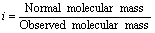In case of association, observed molecular mass being more than the normal, the factor i has a value less than 1. But in case of dissociation, the Van’t Hoff’s factor is more than 1 because the observed molecular mass has a lesser value than the normal molecular mass. In case there is no dissociation the value of ‘i’ becomes equal to one.

Since colligative properties are inversely proportional to molecular masses, the Van’t Hoff’s factor may also be written as,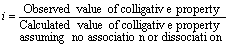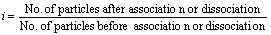Introduction of the Van’t Hoff factor modifies the equations for the colligative properties as follows,

Relative lowering of vapour pressure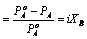Elevation of boiling point,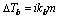Depression in freezing point,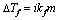Osmotic pressure,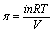;From the value of ‘i’, it is possible to calculate degree of dissociation or degree of association of substance.

Degree of dissociation (a): It is defined as the fraction of total molecules which dissociate into simpler molecules or ions.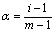; m= number of particles in solution

Degree of association (a): It is defined as the fraction of the total number of molecules which associate or combine together resulting in the formation of a bigger molecules.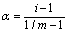; m = number of particles in solution.

## NEET & AIIMS Exam Sample Papers

 AIIMS SAMPLE PAPERS View More NEET SAMPLE PAPERS View More